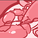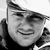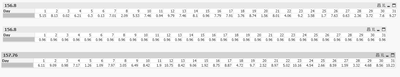Announcements
You can succeed best and quickest by helping others to succeed. Join the conversation.
cancel
Showing results for
Did you mean:Contributor III

## Set Analysis in pivot question

Hello,

Since i dont know how to explain this comprehensively i just try to give an example which should breakdown my problem in an easy way.

I have Sum(sales) for every day of a month in the actual select month in a pivot table. Now i want to add the sum(sales) of a specific day to every other day in the month. The amount can differ and is only available after its acutally calculated in the pivot table.

i.e. Sum(Sales) for the 15th October is 20. This is caclucalated dynamicly daily and can differ each load. Now i want add this 20 to every other in the month like:

sum(sales)+ sum(sales<15th october>) so if sales on 1st is 10 it would 10+20.

When i use set analysis , the pivot table will ignore the second part of the statement on any other day except the 15th , which seems logical, but how can i write the expression that it adds it to every day nevertheless?

is this possbile in a pivot, or do this need to be addressed in the load statement. I tried different combination of aggr/rangesum, but somehow it never works as intended.  In general i want to calculate attrition and  therefore want to refer to a specific day by demand around which i would base the other calculations in the pivot

Thanks

1 Solution

Accepted SolutionsMVP

You might be helped by using total in your second sum () like this.

sum(sales)+ sum( TOTAL  sales<15th october>)

3 RepliesMVP

You might be helped by using total in your second sum () like this.

sum(sales)+ sum( TOTAL  sales<15th october>)Master

somewhat in line with what Vegar suggested, but with some preconditions:
assuming that you have a Month that the user selects to preselect all the DAYS in the month; + have a DAY field which is day(YourDate) - so you can use this in your set analysis.

then in your pivot, use Day as the dimension.  for expression, use
sum(Amount) + sum(total {<Day=15>}Amount)

the first chart shows sum(Amount), 2nd chart shows sum(total {<Day=15>}Amount), 3rd shows the final expressionContributor III
Author

it works, thx a lot guys.Community Browser